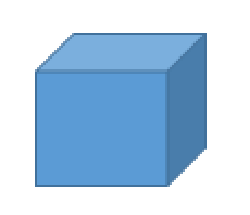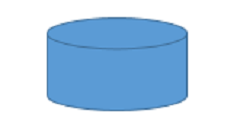# 3-D Shapes: Lesson for Kids

Instructions:

question 1 of 3

### 3 dimensional shapes are made up of what characteristics?

Create Your Account To Take This Quiz

As a member, you'll also get unlimited access to over 79,000 lessons in math, English, science, history, and more. Plus, get practice tests, quizzes, and personalized coaching to help you succeed.

Try it risk-free for 30 days. Cancel anytime

### 1. How many vertices does this 3-dimensional shape have?### 2. How many faces does this 3-dimensional shape have?Create your account to access this entire worksheet
Quizzes, practice exams & worksheets
Certificate of Completion
Create an account to get started

This quiz will ask you several questions about three-dimensional (3-D) shapes. You'll need to know what 3-D shapes are made of and answer four practice problems that use pictures of 3-D shapes.

## Quiz & Worksheet Goals

• Know the characteristics that make up a three-dimensional shapes
• Count the amount of vertices in a 3-D shape
• Determine how many faces a 3-D shape has
• Recognize the number of edges in an example 3-D shape

## Skills Practiced

You'll get practice using the following skills:

• Information recall - remember what you know about the characteristics that make 3-D shapes
• Reading comprehension - take away important information from the three-dimensional shapes lesson
• Problem solving - use your knowledge to solve 3-D shape practice problems

Review the lesson called 3-D Shapes: Lesson for Kids to learn more about these shapes. The lesson helps students:

• Define three-dimensional shapes
• See visual examples of 3-D shapes
• Learn the names of different types of 3-D shapes
Final ExamMath for Kids
Status: Not Started
Chapter ExamGeometry for Elementary School
Status: Not Started

Support